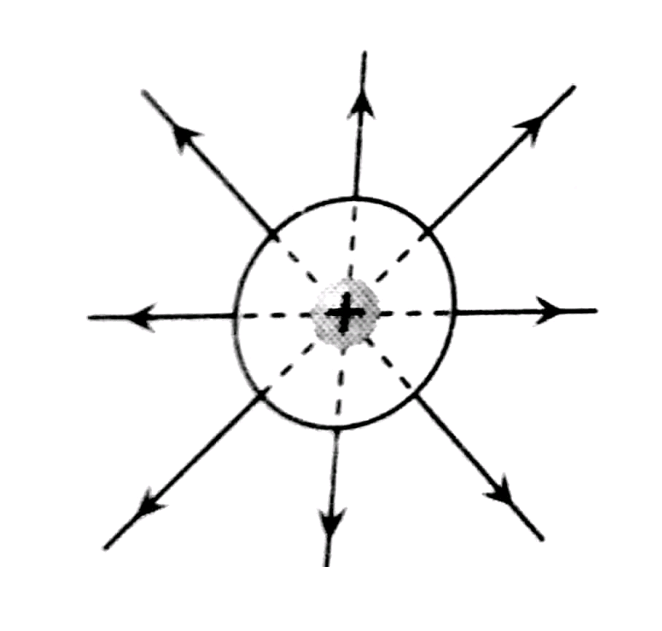Q

# Solve this problem The figure shows the lines of constant potential in a region in which an electric field is present. The magnitude of electric field is maximum at

The figure shows the lines of constant potential in a region in which an electric field is present. The magnitude of electric field is maximum at• Option 1)

A

• Option 2)

B

• Option 3)

C

• Option 4)

Equal at A, B and C

140 Views

As we learned

For Positive charge -

Electric field line comes out.

- whereinSince lines of force are denser at B hence electric field is maximum at B

Option 1)

A

Option 2)

B

Option 3)

C

Option 4)

Equal at A, B and C

Exams
Articles
Questions